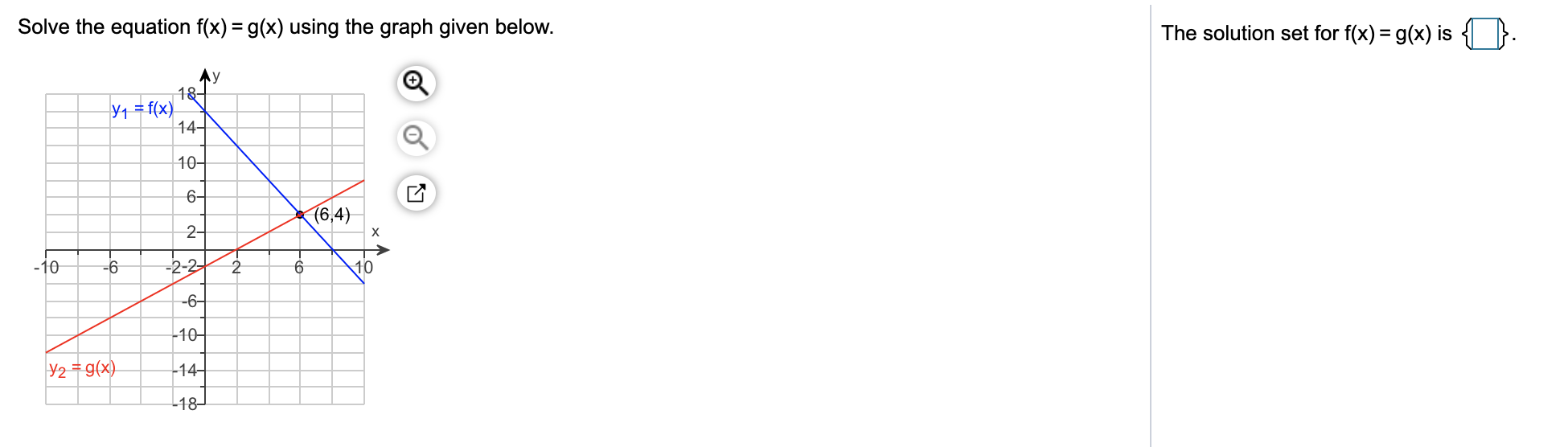# Solve the equation f(x) = g(x) using the graph given below.The solution set for f(x) = g(x) isAy18-Y1 = f(x)14-10-6-(6,4)2--2-2-10-610-6–-10-Y2 = g(x)-14-18-

Question
1 viewshelp_outlineImage TranscriptioncloseSolve the equation f(x) = g(x) using the graph given below. The solution set for f(x) = g(x) is Ay 18- Y1 = f(x) 14- 10- 6- (6,4) 2- -2-2 -10 -6 10 -6– -10- Y2 = g(x) -14- 18- fullscreen
check_circle

Step 1

Given graph is

Step 2

When the graphs of y=f(x) and y=g(x) intersect, both graphs have exactly same value of x and y. Thus The solutio...

### Want to see the full answer?

See Solution

#### Want to see this answer and more?

Solutions are written by subject experts who are available 24/7. Questions are typically answered within 1 hour.*

See Solution
*Response times may vary by subject and question.
Tagged in

### Algebra